# Magnetic Effects of Electric Current:

The flow of electric current constitutes many effects. One of them is heating effect of electricity which we learnt in the electricity chapter. Now another major effect of the electricity we are going to study in this chapter is magnetic effects of electric current. We can find its presence by keeping a magnetic compass near the wire in which current is flowing. You will observe the deflection in the needle of magnetic compass. This proves that electricity shows magnetic effect.

## Magnet: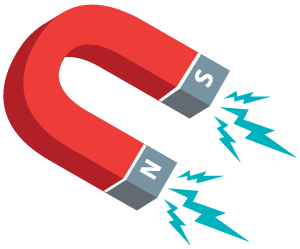• A substance that attracts iron or iron like substance (iron, steel, cobalt and nickel) towards itself is said to be magnet.
• Magnet has two poles – North pole and South pole.
• When a magnet is hanged freely in the air it skips in direction of the north-south pole of the earth.
• If we break magnet in parts then each individual parts act as a magnet.

## Magnetic Field:

• The region around the magnet where the force of attraction or repulsion can be experienced is said to be magnetic field.
• Magnetic field has both magnitude as well as direction, thus, is a vector,
• We measure magnetic field in Tesla or Weber/m2.

## Magnetic field lines: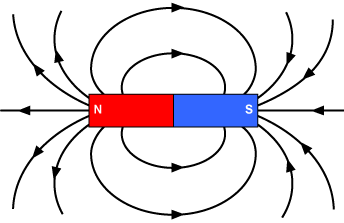• The magnetic field lines are a visual tool used to represent magnetic fields. It is also said as magnetic lines of force.
• It originates from the north pole of the magnet and externally it subtends to the south pole.
• The direction of magnetic field lines outside the magnet is from north to south but inside the magnet it is from south to north pole.
• The direction at a point is defined by drawing a tangent at that point.
• The magnetic field lines are closed curves.
• More closer the field lines more will be the field strength. The magnetic field is stronger at poles.
• No two magnetic field lines intersect.

## Right Hand Thumb Rule:• Imagine that we are holding a current-carrying straight conductor in our right hand such that the thumb points towards the direction of current.
• Then wrapping of our fingers will give the direction of magnetic field.

## Magnetic field due to a current carrying conductor:

• An electric current through a metallic conductor produces magnetic field around it.
• We can determine the direction of magnetic field due to a current carrying conductor by right hand thumb rule.
• The magnetic field produced by a current-carrying straight wire depends inversely on the distance from it.
• We can determine the direction of magnetic field due to a current carrying circular conductor by right hand rule. As at the centre of the circular loop the magnetic field lines adds up to form a straight line.
• A coil which contains many circular turns of insulated copper wire wrapped closely in the shape of a cylinder is called a solenoid.
• The pattern of magnetic field in solenoid is similar as that of a bar magnet.
• One end of the solenoid has the characteristics of magnetic north pole, while the other behaves as the south pole.
• Inside the solenoid the field lines are in the form of parallel straight lines. The magnetic field is uniform inside the solenoid.
• Inside the solenoid, a strong magnetic field is produced which can be used to magnetise a piece of magnetic material, like soft iron, when placed inside the coil. The magnet so formed is called an electromagnet.

## Difference between electromagnet and Permanent magnet:

 Electromagnet Permanent Magnet It is a temporary magnet. Its oviously permanent. It can be demagnetized easily. It can’t be easily demagnetized. The strength of electromagnet  can be varied. The strength of permanent magnet  is fixed. Polarity can be reversed. Polarity can’t be reversed. Generally strong magnet. Generally weak magnet.

## Force on a current carrying conductor in a magnetic field:

• The magnetic field also exerts equal and opposite force on a current carrying conductor.
• When the direction of current through the conductor is reversed, the direction of force is also reversed.

## Fleming’s left-hand rule: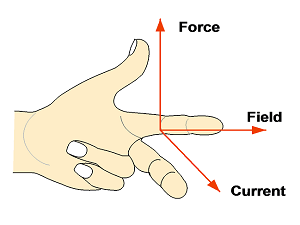• According to Fleming’s Left-Hand Rule, if we Stretch the thumb, forefinger and middle finger of left hand mutually perpendicular to each other.
• If forefinger is placed in the direction of magnetic field and central finger in the direction of current, then the thumb gives the direction of force acting on the conductor.
• Electric current, Magnetic field and Force have same directions as the three mutually perpendicular axes, i.e. x, y and z-axes.
• Some devices like electric motor, electric generator, loudspeakers, microphones and measuring instruments make use of current carrying conductors.

## Applications of Fleming’s left-hand rule:

There are several applications of Fleming’s left hand rule. Some of them are:

### Electric Motor: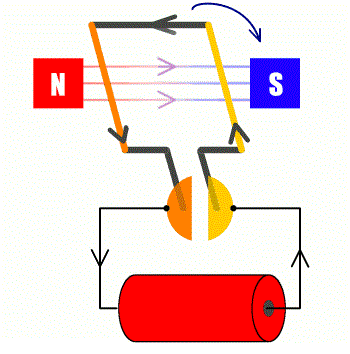• The device which converts electrical energy to mechanical energy is said to be Electric Motor.
• In it, a rectangular coil is suspended between the north and south poles of a magnetic field.
• Commutator (a device which reverses the direction of flow of electric current through a circuit) is connected to the electric supply to the coil.
• When current is passed to the coil of the electric motor, because of the magnetic field it gets deflected.
• After reaching the halfway, the split ring which acts as commutator reverses the direction of flow of electric current.
• When the direction of current reverses the direction of forces acting on the coil also gets reversed.
• The change in direction of force pushes the coil, which supports it to move another half turn.
• Finally, one rotation around the axle of the coil completes. Continuation of this process keeps the motor in rotation motion.

## Electromagnetic Induction: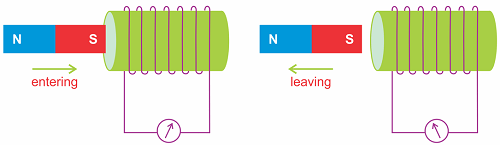• When either a conductor placed in a changing magnetic field or a conductor moving through a stationary magnetic field, there induces current inside the conductor.
• This process of induction of current in the conductor (or causes the production of a voltage across the conductor) is said to be Electromagnetic Induction.

## Flemings Right hand rule: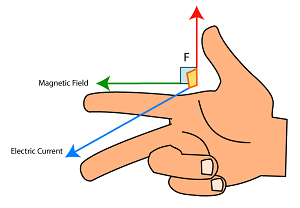• This rule is used to find the direction of induced current.
• According to Fleming’s Right-Hand Rule, if we Stretch the thumb, forefinger and middle finger of Right hand mutually perpendicular to each other.
• If forefinger is placed in the direction of magnetic field and thumb in the direction of motion of conductor then central finger gives the direction of current.

### Electric Generator:

• The device which converts mechanical energy to electrical energy is said to be Electric Generator.
• There are two types of electric generators:
(i) C Generator (ii) D.C Generator
• Electric generator works on the principal of electromagnetic induction.
• There is of a rotating rectangular coil placed between the two poles of a permanent magnet.
• The two ends of this coil are connected to the two rings having insulated inner surface which are internally attached to an axle.
• The axle may be mechanically rotated from outside to rotate the coil inside the magnetic field.
• When the axle is rotated, Currents are induced.
• Larger number of turns in the coil larger will be the amount of induced current.
• By applying Flemings right hand rule we can find the direction of induced current in the rectangular coil.
• The direction of induced current is changed in every half rotation.
• Such a current, which changes direction after equal intervals of time, is alternating current (AC). This device is called an AC generator.
• Such a current, which doesn’t change its direction with respect to time is called Direct Current (DC).
• To get DC we use split ring type commutator.

## Difference between DC (Direct Current) and AC (Alternating current):

 Direct Current Alternating Current In DC, the flow of electric charge is only in one direction. In AC, the flow of electric charge periodically reverses direction. Voltage of DC cannot travel very far until it begins to lose energy. AC is safe to transfer over longer city distances and can provide more power. Magnitude of DC is constant over time. Magnitude of AC varies with time. The frequency of DC is zero. The frequency of AC is 50Hz in India.

## Domestic Electric Circuit:

• Electric supply in our homes said to be mains or main supply.
• It has two wires, one is live wire (positive) which is covered with red insulation whereas the other is neutral wire (negative) having black or other colour insulation.
• The other wire is with green insulation is earth wire.
• The earth wire connected to a metal plate deep in the earth near the house which saves us from getting shock from appliances which has metallic bodies (metallic bodies is connected to earth wire).
• In India, the potential difference is 220 V.
• Each appliance has its own switch which allows its own on or off.
• When Live wire comes in direct contact with the neutral wire accidently then it is said to be short circuit. Thus resistance of the circuit decreases which causes overloading.
• Overloading is mainly caused when current drawn in the circuit is more then the required current to the appliance or by connecting too many appliances to a single socket.

## Electric Fuse:• Electric fuse is a short piece of wire made of a material of high resistance and low melting point.
• A fuse is a safety device in a circuit prevents damage to the appliances and the circuit due to overloading.
• The Joule heating that takes place in the fuse melts it to break the electric circuit thus prevents the damage.